# 9. Solve the system by back substitution. -2y - 12z-4w16 -2z-2w-4 The solution set is (...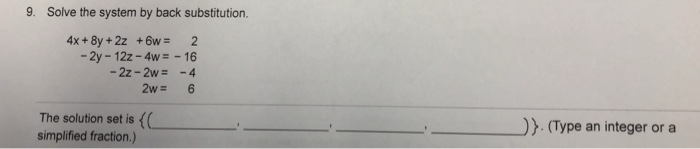9. Solve the system by back substitution. -2y - 12z-4w16 -2z-2w-4 The solution set is ( simplified fraction.) D). (Type an integer or a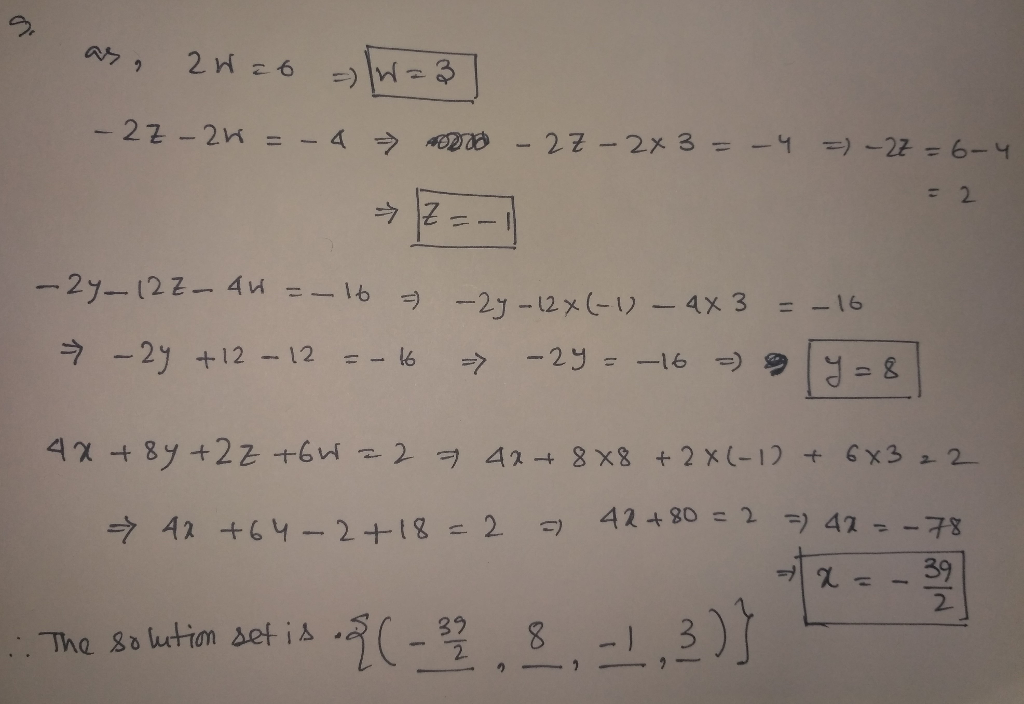##### Add Answer to: 9. Solve the system by back substitution. -2y - 12z-4w16 -2z-2w-4 The solution set is (...
Similar Homework Help Questions
• ### Use row operations on an augmented matrix to solve the following system of equations. X+ y-...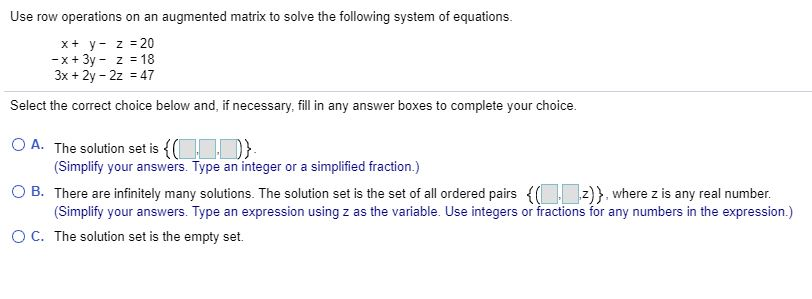Use row operations on an augmented matrix to solve the following system of equations. X+ y- z = 20 - x + 3y - 2 = 18 3x + 2y - 2z = 47 Select the correct choice below and, if necessary, fill in any answer boxes to complete your choice. O A. The solution set is {C}. (Simplify your answers. Type an integer or a simplified fraction.) OB. There are infinitely many solutions. The solution set is the set...

• ### Solve the system. - 5X - Y = 7 y-2z = 5 x - 2y +...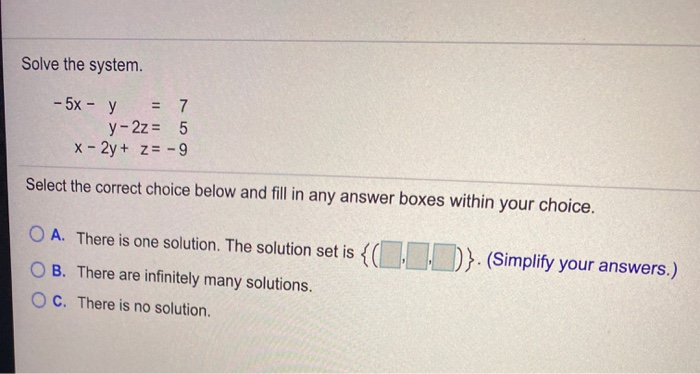Solve the system. - 5X - Y = 7 y-2z = 5 x - 2y + z = -9 Select the correct choice below and fill in any answer boxes within your choice. O A. There is one solution. The solution set is {(1,1))}. (Simplify your answers.) OB. There are infinitely many solutions. OC. There is no solution.

• ### Use back-substitution to solve the system of linear equations. (If there is no solution, enter NO...

Use back-substitution to solve the system of linear equations. (If there is no solution, enter NO SOLUTION. If the system is dependent, set z = a and solve for x and y in terms of a.) 10x − 4y + z = 47 −y + z = 7 z = 2 (x, y, z) =

• ### Use back-substitution to solve the system of linear equations. (If there is no solution, enter NO...

Use back-substitution to solve the system of linear equations. (If there is no solution, enter NO SOLUTION. If the system is dependent, set z = a and solve for x and y in terms of a.) 6x − 5y + z = 52 −y + z = 11 z = 6 (x, y, z) =

• ### Solve the equation after making an appropriate substitution, *4-6x² +8=0 Determine the appropriate substitution using the...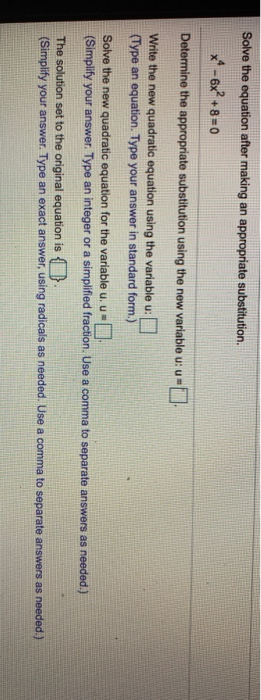Solve the equation after making an appropriate substitution, *4-6x² +8=0 Determine the appropriate substitution using the new variable u: U Write the new quadratic equation using the variable u: (Type an equation. Type your answer in standard form.) Solve the new quadratic equation for the variable u. u= (Simplify your answer. Type an integer or a simplified fraction. Use a comma to separate answers as needed.) The solution set to the original equation is a. (Simplify your answer. Type an...

• ### How do I solve the following system by substitution 5x-2y=-5 y-5x=3 The solution for this system is =>

How do I solve the following system by substitution 5x-2y=-5 y-5x=3 The solution for this system is =>

• ### Write the solution set of the given homogeneous system in parametric vector form. 4x1 + 4x2...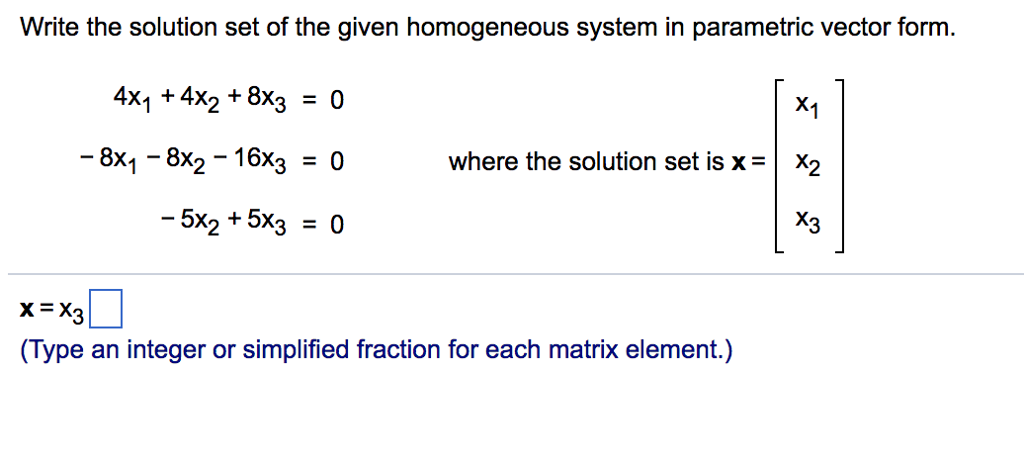Write the solution set of the given homogeneous system in parametric vector form. 4x1 + 4x2 + 8x3 = 0 8x1-8x2-16x3-0 5x2 + 5x3 = 0 X1 where the solution set is x- X2 X3 x=x3 (Type an integer or simplified fraction for each matrix element.)

• ### 1. Solve the following system of equations using Gauss-Jordan elimination. 3x - 2y +4z=3 2x +2y-2z=4...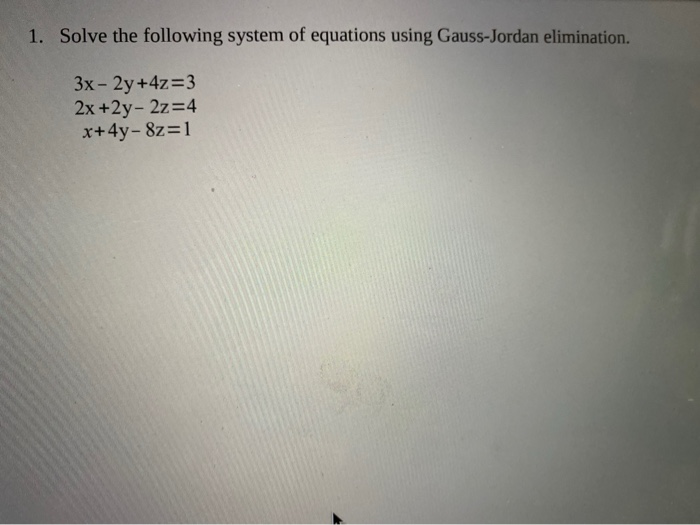1. Solve the following system of equations using Gauss-Jordan elimination. 3x - 2y +4z=3 2x +2y-2z=4 x+4y- &z=1

• ### Solve the system using either Gaussian elimination with back-substitution or Gauss-Jordan elimination. (If there is no...

Solve the system using either Gaussian elimination with back-substitution or Gauss-Jordan elimination. (If there is no solution, enter NO SOLUTION. If the system has an infinite number of solutions, set y = t and solve for x in terms of t.) −3x + 5y = −35 3x + 4y = −1 4x − 8y = 52

• ### Systems of Equations: 3x + y = 6 2x-2y=4 Substitution: Elimination: Solve 1 equation for 1...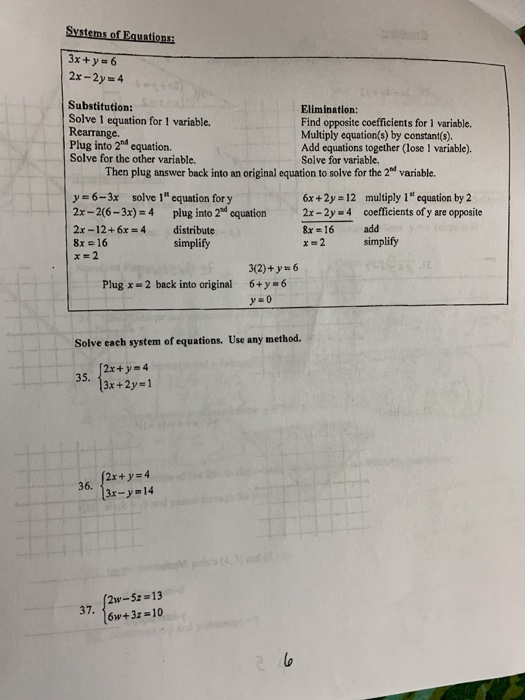Systems of Equations: 3x + y = 6 2x-2y=4 Substitution: Elimination: Solve 1 equation for 1 variable. Find opposite coefficients for 1 variable. Rearrange. Multiply equation(s) by constant(s). Plug into 2nd equation Add equations together (lose 1 variable). Solve for the other variable. Solve for variable. Then plug answer back into an original equation to solve for the 2nd variable. y = 6 -- 3x solve 1" equation for y 6x +2y = 12 multiply 1" equation by 2 2x...

Free Homework App